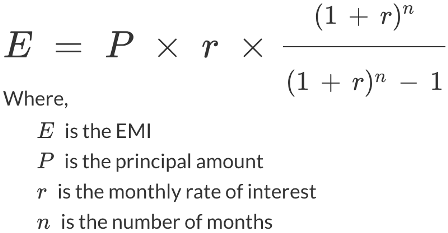### What is the meaning of EMI?

EMI refers to the ‘Equated Monthly Installment’ which is the amount you will pay to a loan provider on a specific date each month till the loan is repaid in full. The EMI comprises of the principal and interest components which are structured in a way that in the initial years of your loan, the interest component is much larger than the principal component, while towards the latter half of the loan, the principal component is much larger.

### Formula to calculate EMI### How to Calculate EMI Using Mathematical Formula.

EMI = [P x R x (1+R)^N]/[(1+R)^ (N-1)],

Here in this formula, the variables stand for:

EMI is the equated monthly instalment

P is the principal or the amount that is borrowed as a loan

R is the rate of interest that is levied on the loan amount (the interest rate should be a monthly rate)

N is the tenure of repayment of the loan or the number of monthly instalments that you will pay (tenure should be in months)

This is the same formula an EMI calculator uses to provide you with the correct EMI payable within seconds.

Let us consider an example to understand EMI calculations in a better way,

For instance, you have taken a personal loan of Rs. 2 lakhs for 2 years at an interest of 20 % p.a.

Firstly, we need to convert the annual interest rate into a monthly rate and the tenure into months.

To calculate the monthly interest rate, we divide the annual interest rate by the number of months in a year, i.e. 12, so monthly 20/12 = 1.66% per month

The 2-year loan tenure must also be converted into months before integrating into the formula i.e. 24 months

Now we have the three variables with us which we can integrate into the formula as follows:

EMI = [P x R x (1+R)^N]/[(1+R)^N-1]

EMI= [2,00,000 x 1.66/100 x (1+1.66/100) ^ 24 / [(1+1.66/100) ^ 24 – 1)

EMI= Rs. 10, 179